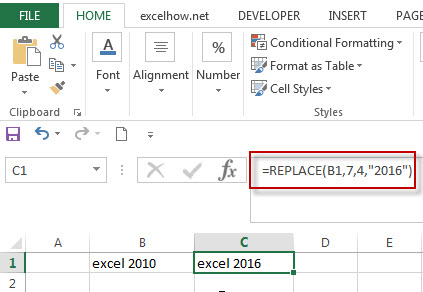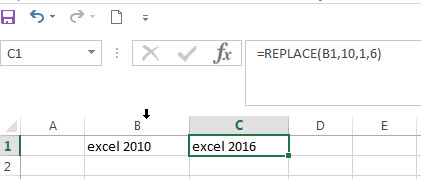# Excel Replace function with numeric values

In excel, how to replace a numeric characters within a text string using another numeric characters. This post will guide you how to use REPLACE function to replace numeric characters using another numeric characters in one text string.

The Excel REPLACE function replaces all or part of a text string with another text string

The syntax of the REPLACE function is as below:

`=REPLACE(old_text, start_num, num_chars, new_text)`

If you want to replace numeric characters that are part of text string with another text string, it can be numeric characters, for example: replacing the numeric characters “2010”of text string “excel 2010” with another number “2016”, you can use the following REPLACE function in excel 2016:

`=REPLACE(B2,7,4,2016)`The returned results of REPLACE function is still a text string, you can see that the text string “excel 2016” be returned.

You can also just replace one or more digits within a number, we can use another REPLACE formula to reflect the above request.

```=REPLACE(B1,10,1,6)
```In the above example, the REPLACE Function will only replace one digit that are part of numeric characters with a new numeric value “6”. The text string “excel 2016” will be returned.

### Related Functions

• Excel Replace function
The Excel DATE function returns the serial number for a date.The syntax of the DATE function is as below:= DATE (year, month, day) …

Sidebar